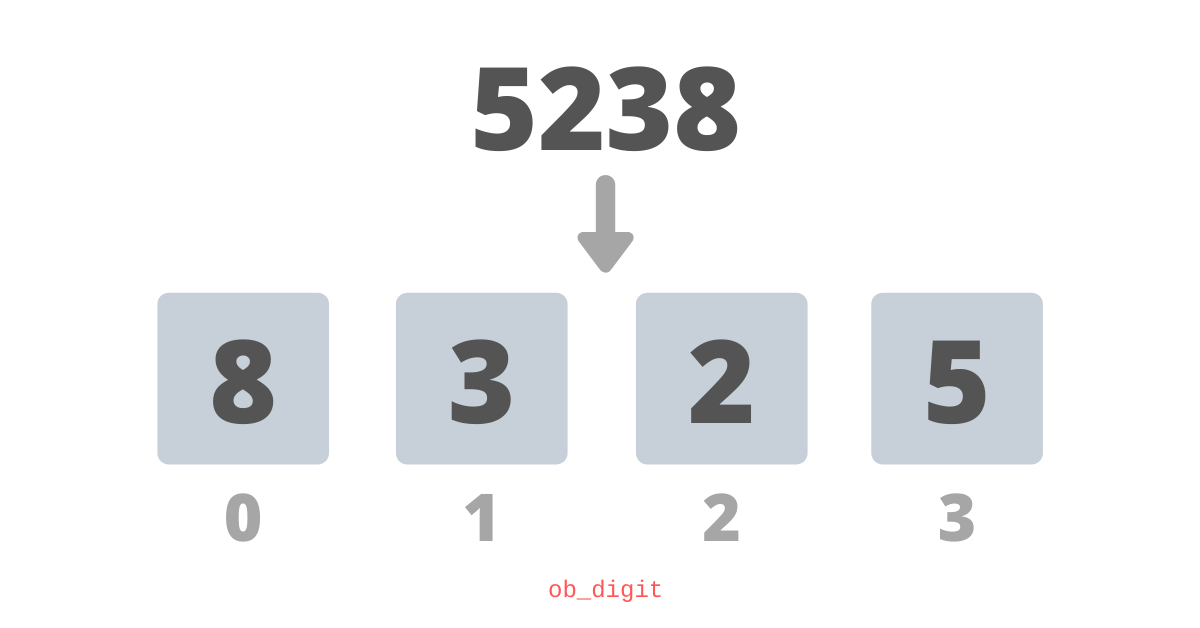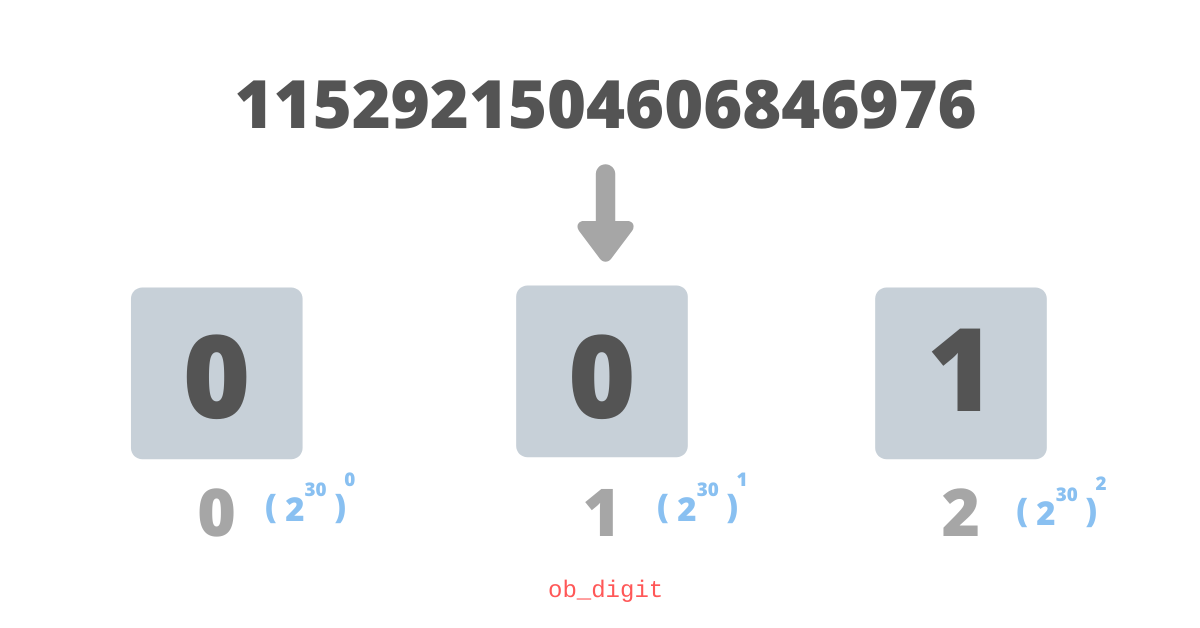# Python supports integers of infinite length, but how?

When you code in a low-level language like C, you worry about picking the right data type and qualifiers for your integers; at every step, you need to think if `int` would suffice or should you go for a `long` or even higher to a `long double`. But while coding in python, you need not worry about these “trivial” things because python supports integers of arbitrary size.

In C, when you try to compute 220000 using builtin `powl` function it gives you `inf` as the output.

``````#include <stdio.h>
#include <math.h>

int main(void) {
printf("%Lf\n", powl(2, 20000));
return 0;
}

\$ ./a.out
inf``````

But for python, it is a piece of cake 🎂

``````>>> 2 ** 20000
39802768403379665923543072061912024537047727804924259387134 ...
...
... 6021 digits long ...
...
6309376``````

Python must be doing something beautiful internally to support integers of arbitrary sizes and today we find out what’s under the hood!

# Representation and definition

An integer in Python is a C struct defined as following

``````struct _longobject {
digit ob_digit;
};``````

`PyObject_VAR_HEAD` is a macro that expands into a `PyVarObject` that has the following structure

``````typedef struct {
PyObject ob_base;
Py_ssize_t ob_size; /* Number of items in variable part */
} PyVarObject;``````

Other types that has `PyObject_VAR_HEAD` are

• `PyBytesObject`
• `PyTupleObject`
• `PyListObject`

This indicates that an integer, just like a `tuple` or a `list`, is variable in length and this is our first insight into how it could support gigantically long integers. The `_longobject` after macro expansion could be roughly seen as

``````struct _longobject {
PyObject ob_base;
Py_ssize_t ob_size; /* Number of items in variable part */
digit ob_digit;
};``````

These are some meta fields in the `PyObject` struct, used for reference counting (garbage collection), but that we would require a separate article. The field that we will focus on is `ob_digit` and to some extent `ob_size`.

### Decoding `ob_digit`

`ob_digit` is an array of type `digit`, typedef’ed from `uint32_t`, statically allocated to length `1`. Since it is an array, `ob_digit` primarily is a `digit *`, pointer to `digit`, and hence if required could be malloced to any length. This makes it possible for python to represent and handle gigantically long integers.

Generally, In low-level languages like C, the precision of integers is limited to 64-bit, but Python implements Arbitrary-precision integers. Since Python 3 all integers are represented as a bignum and these are limited only by the available memory of the host system.

### Decoding `ob_size`

`ob_size` holds the count of elements in `ob_digit`. To be more efficient while allocating the memory to array `ob_digit`, python over-provisions and then relies on the value of `ob_size` to determine the actual number of elements held int the array.

# Storage

A naive way to store an integer digit-wise is by actually storing a decimal digit in one item of the array and then operations like addition and subtraction could be performed just like grade school mathematics.

With this approach, a number `5238` will be stored asThis approach is inefficient as we will be using up 32 bits of digit (`uint32_t`) to store a decimal digit that actually ranges only from 0 to 9 and could have been easily represented by mere 4 bits, and while writing something as versatile as python, a core developer has to be more resourceful than this.

So, can we do better? for sure, otherwise, this article should hold no place on the internet. Let’s dive into how python stores a super long integer.

## The pythonic way

Instead of storing just one decimal digit in each item of the array `ob_digit`, python converts the number from base 10 to base 230 and calls each of element as `digit` which ranges from 0 to 230 - 1.

In the hexadecimal number system, the base is 16 ~ 24 this means each “digit” of a hexadecimal number ranges from 0 to 15 of the decimal system. Similarly for python, “digit” is in base 230 which means it will range from 0 to 230 - 1 = 1073741823 of the decimal system.

This way python efficiently uses almost all of the allocated space of 32 bits per digit and keeps itself resourceful and still performs operations such as addition and subtraction like grade school mathematics.

Depending on the platform, Python uses either 32-bit unsigned integer arrays with 30-bit digits or 16-bit unsigned integer arrays with 15-bit digits. It requires a couple of bits to perform operations that will be discussed in some future articles.

### Example: 1152921504606846976

As mentioned, for Python a “digit” is base 230 hence if you convert `1152921504606846976` into base 230 you get `100`

1152921504606846976 = 1 * (230)2 + 0 * (230)1 + 0 * (230)0

Since `ob_digit` persists it least significant digit first, it gets stored as `001` in 3 different digits.

The `_longobject` struct for this value will hold

• `ob_size` as `3`
• `ob_digit` as `[0, 0, 1]`I have created a demo REPL that will output the way python is storing integers internally and also has reference to struct members like `ob_size`, `ob_refcount`, etc.

# Operations on super long integers

Now that we have a fair idea on how python supports and implements arbitrary precision integers its time to understand how various mathematical operations happen on them.

Integers are persisted “digit-wise”, this means the addition is as simple as what we learned in the grade school and python’s source code shows us that this is exactly how it is implemented as well. The function named x_add in file longobject.c performs the addition of two numbers.

``````...
for (i = 0; i < size_b; ++i) {
carry += a->ob_digit[i] + b->ob_digit[i];
carry >>= PyLong_SHIFT;
}
for (; i < size_a; ++i) {
carry += a->ob_digit[i];
carry >>= PyLong_SHIFT;
}
z->ob_digit[i] = carry;
...``````

The code snippet above is taken from `x_add` function and you could see that it iterates over the digits and performs addition digit-wise and computes and propagates carry.

Things become interesting when the result of the addition is a negative number. The sign of `ob_size` is the sign of the integer, which means, if you have a negative number then `ob_size` will be negative. The absolute value of `ob_size` will determine the number of digits in `ob_digit`.

## Subtraction

Similar to how addition is implemented, subtraction also happens digit-wise. The function named x_sub in file longobject.c performs subtraction of two numbers.

``````...
for (i = 0; i < size_b; ++i) {
borrow = a->ob_digit[i] - b->ob_digit[i] - borrow;
borrow >>= PyLong_SHIFT;
borrow &= 1; /* Keep only one sign bit */
}
for (; i < size_a; ++i) {
borrow = a->ob_digit[i] - borrow;
borrow >>= PyLong_SHIFT;
borrow &= 1; /* Keep only one sign bit */
}
...``````

The code snippet above is taken from `x_sub` function and you could see how it iterates over the digits and performs subtraction and computes and propagates burrow. Very similar to addition indeed.

## Multiplication

Again a naive way to implement multiplication will be what we learned in grade school math but it won’t be very efficient. Python, in order to keep things efficient implements the Karatsuba algorithm that multiplies two n-digit numbers in O( nlog23) elementary steps.

The algorithm is slightly complicated is out of the scope of this article but you can find its implementation in k_mul and k_lopsided_mul functions in file longobject.c.

## Division and other operations

All operations on integers are defined in the file longobject.c and it is very simple to locate and trace each one. Warning: it will take some time to understand each one in detail so grab some popcorn before you start skimming.

# Optimization of commonly-used integers

Python preallocates small integers in a range of -5 to 256. This allocation happens during initialization and since we cannot update integers (immutability) these preallocated integers are singletons and are directly referenced instead of reallocating. This means every time we use/creates a small integer, python instead of reallocating just returns the reference of preallocated one.

This optimization can be traced in the macro `IS_SMALL_INT` and the function get_small_int in longobject.c. This way python saves a lot of space and computation for commonly used integers.

This essay is heavily inspired, and to some extent copied, from Artem Golubin’s post - Python internals: Arbitrary-precision integer implementation. In case you want a detailed deep dive on CPython Integers or CPython Internals in general, I recommend you checkout the CPython Internal Series by Artem Golubin.

Thank you Artem Golubin for all the amazing CPython Internal articles. This essay is heavily inspired, and to some extent copied, from Artem Golubin’s post - Python internals: Arbitrary-precision integer implementation. In case you want a detailed deep dive on CPython Integers or CPython Internals in general, I recommend you check out the CPython Internal Series by Artem Golubin.

Thank you Artem Golubin for all the amazing CPython Internal articles.

### Courses

Super practical courses, with a no-nonsense approach, are designed to spark engineering curiosity and help you ace your career.

## System Design for Beginners

An in-depth, self-paced, and on-demand course that for early engineers to become great at designing scalable, available, and extensible systems at scale.

## System Design Masterclass

A masterclass that helps experienced engineers become great at designing scalable, fault-tolerant, and highly available systems.

## Redis Internals

A course that helps covers Redis internals by reimplementing its core features like - event loop, serialization protocol, pipelining, eviction, and transactions.## CS newsletter for the curious engineers

If you like what you read subscribe you can always subscribe to my newsletter and get the post delivered straight to your inbox. I write essays on various engineering topics and share it through my weekly newsletter.

Writings and Videos
Legal and Contact